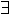Recursive functions in GST
Overview
 Introduction A new "gst-rec" theory is created as a child of "gst-fun". Probably Definitions Definition of the function "recdef" which converts a recursion functional into the function defined by that functional.
 Recursion Theorem Proof Context In this section I will create a decent proof context for recursive definitions, eventually. Listing of Theory gst-rec
Introduction
 A new "gst-rec" theory is created as a child of "gst-fun". Probably
The Theory gst-rec
The new theory is first created, together with a proof context which we will build up as we develop the theory.
 xl-sml open_theory "gst-fun"; force_new_theory "gst-rec"; force_new_pc "gst-rec"; merge_pcs ["xl_cs__conv"] "gst-rec"; set_merge_pcs ["basic_hol", "gst-ax", "gst-fun", "gst-rec"];

Definitions
 Definition of the function "recdef" which converts a recursion functional into the function defined by that functional.
 Introduction The idea here is that a of recursive equation is represented by a functional, which is obtained by abstracting the right hand side of the equation on the name of the function. We want to define
Recursion Theorem
Proof Context
 In this section I will create a decent proof context for recursive definitions, eventually.
Proof Context

 xl-sml commit_pc "gst-rec";

\$Id: recursion.xml,v 1.1.1.1 2000/12/04 17:24:21 rbjones Exp \$